0
article(s) | €
0,00
We have simplified the calculation for you. If you enter the measurement X and the remanence Br of the magnet material there, the software calculates the flux density at point (x).
Example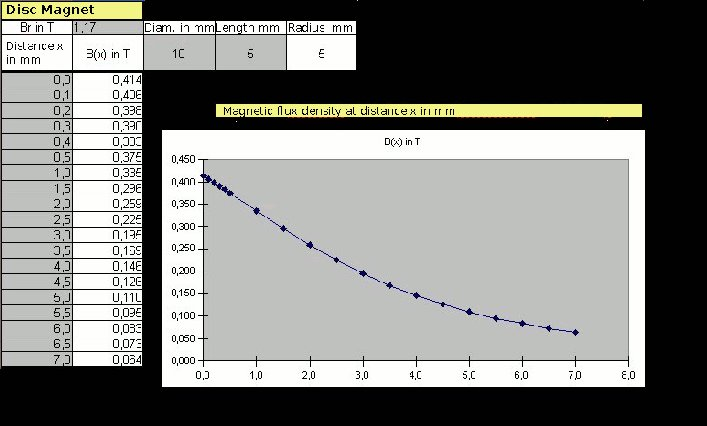Circular disc magnet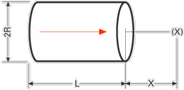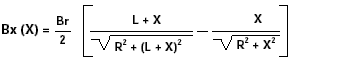Example: How large is the flux density at a distance of 5 mm for a circular disc magnet made of DeltaMagnet (SmCo) 6 Ø x 4 mm (IBS standard type DE64)?

Br of DeltaMagnet from the table = 1000 mT

Result: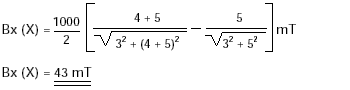Circular disc magnet with iron reflux plate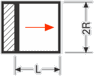1. You can replace L by 2 x L in the formula, if an iron reflux plate is placed on the pole area behind the circular disc magnet. The thickness of the reflux plate should be chosen in such a way that no magnetic saturation is caused.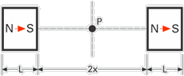2. The value Bx for point P will be doubled, if two identical circular disc magnets (i.e. attractive) are placed parallel to each other.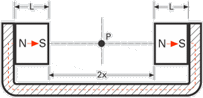3. The value Bx for point P will be doubled (L replaced by 2 x L), if the circular disc magnets placed parallel to each other (shown in Fig. 2.) are connected by an iron hitch.

Square magnet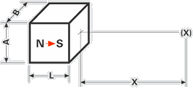Formula to calculate the flux density Bx in distance (X)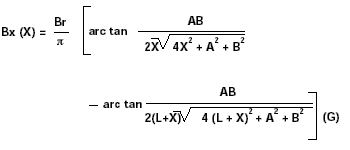The formula is not defined for X=0. The Arcus tangent (arc tan) must be calculated in radian measure.